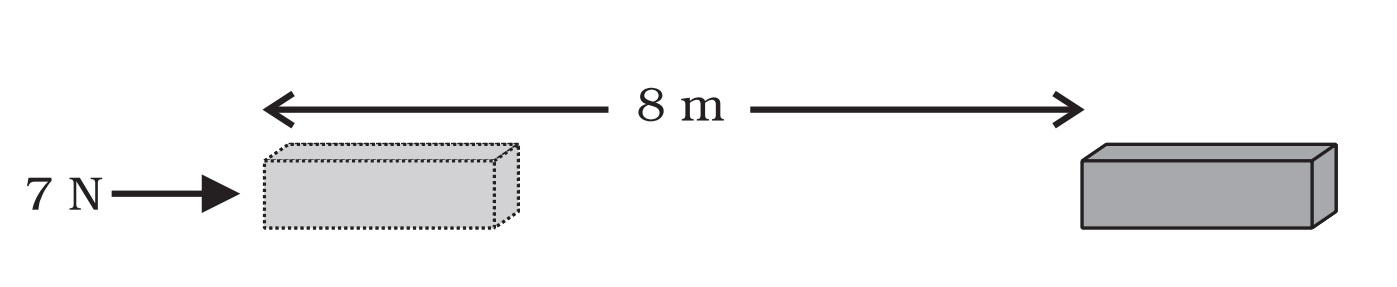Questions from Inside the chapter

Class 9
Chapter 11 Class 9 - Work and Energy

## A force of 7 N acts on an object. The displacement is, say 8 m, in the direction of the force (Fig. 11.3). Let us take it that the force acts on the object through the displacement. What is the work done in this case?

###googletag.cmd.push(function() { googletag.display('div-gpt-ad-1669298377854-0'); });

Force = 7 N

Displacement = 8m

Work done = Force × Displacement

= 7 × 8

= 56 J

Hence, Work done is 56 J

Learn in your speed, with individual attention - Teachoo Maths 1-on-1 Class